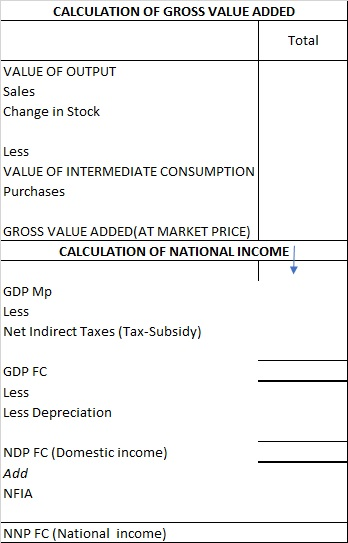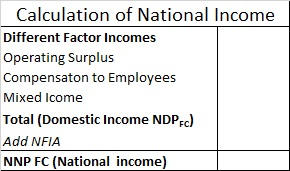Chapter 2 National Income - Part 4 Income Method

Economics Class 12
Macroeconomics

## What is Operating Surplus

It is total of income from property + Income from entrepreneurship

Operating Surplus =Income from Property +Income from Entrepreneurship

Operating Surplus =(Rent + Royalty + Interest) +(Profit)

## How to Calculate Domestic income using Operating Surplus

Domestic income= Rent + Royalty + Interest + Compensation to employees + Profit + Mixed Income

Domestic income= Rent+ Royalty + Interest + Profit + Compensation to employees + Mixed Income

Domestic income=Operating Surplus + Compensation to employees + Mixed Income

Second Formula of Operating Surplus

Operating Surplus=Value of Output-Intermediate Consumption-Net indirect tax-Dep-Compensation to employees-Mixed Income

Explanation

#### How is this formula Derived ?

We know that as per Value Added Method

We derive Domestic Income and National Income as followsHence as per Value Added Method

Value of Output-Intermediate Consumption-Net indirect tax-Dep=NDP FC

Also we have Studied that as per income Method

Income MethodHence

Operating Surplus + Compensation to employees + Mixed Income = NDP FC

NDP FC =Operating Surplus + Compensation to employees + Mixed Income

So we have 2 equations

Value of Output-Intermediate Consumption-Net indirect tax-Dep=NDP FC

NDP FC = Operating Surplus + Compensation to employees +Mixed Income

Putting the value of Second equation in first

Value of Output-Intermediate Consumption-Net indirect tax-Dep=Operating Surplus + Compensation to employees + Mixed Income

Value of Output-Intermediate Consumption-Net indirect tax-Dep-Compensation to employees-Mixed Income=Operating Surplus

Operating Surplus=Value of Output-Intermediate Consumption-Net indirect tax-Dep-Compensation to employees-Mixed Income

Operating Surplus=Value of Output-Intermediate Consumption-Net indirect tax-Dep-Compensation to employees-Mixed Income

(WHATEVER IS NOT GIVEN IN QUESTION,TAKE AS 0)

#### Example 26.

Calculate the Operating Surplus.

 Particulars ₹ in Crores (i) Value of output 70,000 (ii) Purchase of raw material 18,000 (iii) Net indirect tax 3,000 (iv) Wages and salaries 25,000

Operating Surplus=Value of Output-Intermediate Consumption-Net indirect tax-Dep-Compensation to employees-Mixed Income

Operating Surplus = 70000 - 18000 -3000 - 25000

Operating Surplus = 24,000

## What is Operating Surplus

It is total of income from property + Income from entrepreneurship

Operating Surplus =Income from Property +Income from Entrepreneurship

Operating Surplus =(Rent + Royalty + Interest) +(Profit)

hence, if Rent, Royalty, Interest and profit are also given in question

They are not to be taken

#### Question 23

Calculate operating surplus.

 Particulars ₹  in crores (i) Bonus to employees 25 (ii) Mixed income 175 (iii) Profit 100 (iv) Dividend 40 (v) Corporate tax 30 (vi) Rent 80 (vii) Royalty 40 (viii) Interest 130 (ix) Employers' contribution to social security schemes 30

In this Question, we are not given Sales or Intermediate Consumption

So ,we Calculate Operating Surplus using Normal Formula

Operating Surplus =(Rent+ Royalty+ Interest) +(Profit)

Operating Surplus = 80 + 40 + 130 + 100 = 350 Crores

#### Question 24

Calculate the value of operating surplus.

 Particulars ₹ in croses (i) Value of output 800 (ii) Intermediate consumption 200 (iii) Compensation of employees 200 (iv) Indirect taxes 30 (v) Depreciation 20 (vi) Subsidies 50 (vii) Mixed income 100

Sometimes in question

GDP at MP is given instead of Sales and Intermediate Consumption

Sales-Intermediate Consumption=GDP at MP

Now, for calculating Operating Surplus

Operating Surplus=Value of Output-Intermediate Consumption-Net indirect tax-Dep-Compensation to employees-Mixed Income

Operating Surplus=GDP at MP--Net indirect tax-Dep-Compensation to employees-Mixed Income

#### Question 25

Calculate the operating surplus.

 Particulars ₹ in crores (i) Compensation of employees 200 (ii) Indirect taxes 200 (iii) Consumption of fixed capital 100 (iv) Subsidies 50 (v) Gross domestic product at MP 600

Operating Surplus=GDP at MP-Net indirect tax-Dep-Compensation to employees-Mixed Income

Operating Surplus = 600 - 150 -100 - 200

Operating Surplus = 150

#### Question 26

Calculate operating surplus and compensation of employees.

 Particulars ₹ in crores (i) Indirect taxes 250 (ii) Depreciation 200 (iii) Royalty 20 (iv) Profit 200 (v) Subsidies 50 (vi) Gross domestic product at MP 1,800 (vii) Interest 50 (viii) Rent 100 (ix) Net factor income from abroad (-) 40

In this question,

We need to calculate 2 things

Operating Surplus

Compensation to Employees

Step 1- Calculate Operating Surplus

We Calculate Operating Surplus using Normal Formula

Operating Surplus =(Rent + Royalty + Interest) +(Profit)

Operating Surplus = 100+20+50+200 = 370

Step 2-Compensation to employees

for This, we use second formula for Operating Surplus

Operating Surplus=GDP at MP-Net indirect tax-Dep-Compensation to employees-Mixed Income

370 = 1800-200-200- Compensation to Employees-0

Compensation to Emp = 1800-200-200-370

Compensation to Emp = 1030

Learn in your speed, with individual attention - Teachoo Maths 1-on-1 Class